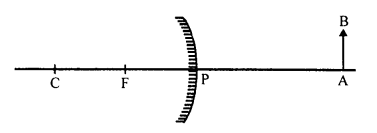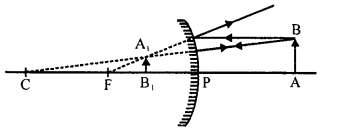# A New Approach to ICSE Physics Part 1 Class 9 Solutions Light

## A New Approach to ICSE Physics Part 1 Class 9 Solutions Light

These Solutions are part of A New Approach to ICSE Physics Part 1 Class 9 Solutions. Here we have given A New Approach to ICSE Physics Part 1 Class 9 Solutions Light.

Unit I
Exercise 1

Question 1.
(a) What do you understand by the following terms?

1. Light
2. Diffused light

(b) By giving one example and one use explain or define

1. Regular reflection
2. Irregular reflection.

(a)
(i) Light : Light is a form of energy which produces in us sensation of seeing.
(ii) Diffused light : Light obtained after reflection from rough surface is known as diffused light.
It is a soft light with neither the intensity nor the glare of direct light. It is scattered and comes from all directions. It does not cause harsh shadows.
(b)
(i) Regular reflection : The phenomenon due to which a parallel beam of light travelling through a certain medium, on striking some polished surface, bounces off from it, as parallel beam, in some other direction is called regular reflection.
For example : Reflection taking place from the objects like looking glass, still water, oil, highly polished metals is regular reflection.
Regular reflection is useful in the formation of images. We can see our face in a mirror only due to regular reflection.
(ii) Irregular reflection or Diffused reflection : The phenomenon due to which a parallel beam of light, travelling through some medium, gets reflected in various possible directions, on striking some rough surface is called irregular reflection.
For example : Reflection taking place from ground, walls, trees, suspended particles in air is irregular reflection.
Use : It helps in the general illumination of places and helps us to see things around us.

Question 2.
By drawing a neat diagram define the following :

1. Mirror
2. Incident ray
3. Reflected ray
4. Angle of incidence
5. Angle of reflection
6. Normal

1. Mirror is a highly polished and smooth surface which reflects almost the entire light falling on it. A plane mirror is made by silvering one side of a glass plate as shown in figure.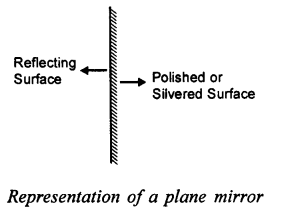2. Incident ray : The light ray striking a reflecting surface is called the incident ray.
3. Reflected ray : The light ray obtained after reflection from the surface, in the same medium in which the incident ray is travelling, is called the reflected ray.
4. Angle of incidence : The angle which the incident ray makes with the normal at the point of incidence is called the angle of incidence. It is denoted by the letter “i”.
5. Angle of reflection : The angle which the reflected ray makes with the normal at point of incidence, is called the angle of reflection. It is denoted by the letter “r”.6. Normal : The perpendicular drawn at the point of incidence, to the surface of mirror is called normal.

Question 3.
State the laws of reflection.
Laws of reflection :

1. The incident ray, the reflected ray and the normal ray at the point of incidence, lie in the same plane.
2. The angle of incidence i is equal to the angle of reflection r i.e. ∠i = ∠r.

Question 4.
A ray of light strikes a plane mirror, such that angle with the mirror is 20°. What is value of angle of reflection? What is the angle between the incident ray and the reflected ray?
∵ Light ray makes an angle of 20° with the mirror
∴ ∠ABM = 20°
Angle of incidence = ∠i = 90° – 20°
∠i = 70°
∵ ∠i = r ∴ ∠r = 70°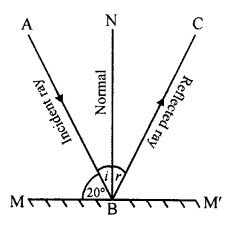Angle between incident ray and reflected ray = ∠i + ∠r = 70°+ 70°= 140°

Question 5.
Prove experimentally that images are formed as far behind in a plane mirror as the object is in front of it.
Consider an object‘O’ situated in front of a plane mirror MM,. A ray of light which starts from point ‘O’ perpendicularly, is reflected back along the same path (See figure).
However, another ray which moves along OB is reflected along BC, obeying the laws of reflection, such that BN is the normal. Produce OA and CB backward, such that they meet at point I. Then ‘I’ is image of ‘O’.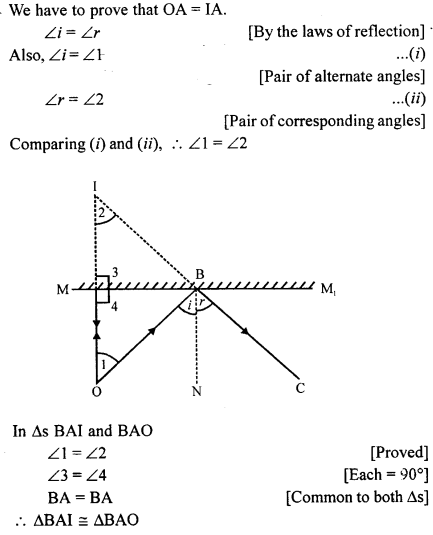Thus, in particular OA = IA.

Question 6.
Prove geometrically that when plane mirror turns through a certain angle, the reflected ray turns through twice the angle.
Consider a ray of light AB, incident on plane mirror in position MM’, such that BC is the reflected ray and BN is the normal.
∠ABM = ∠CBN = ∠i
∠ABC = 2 ∠i …(i)
Let the mirror be rotated through an angle ‘0’ about point B, such that M1M1 is the new position of the mirror and BN1 is the new position of normal. As the position of the incident ray remains same, therefore new angle of the incidence is ∠ABN1 whose magnitude is (i + θ). Let BD be the reflected ray, such that ∠DNB1 is the new angle of reflection.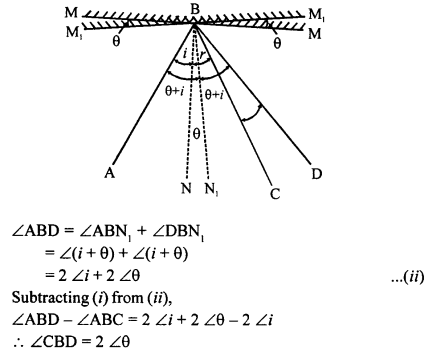Thus, for a given incident ray, if the plane mirror is rotated through a certain angle, then the reflected ray rotates through twice the angle.

Question 7.

(a) What do you understand by the term lateral inversion?
(b) A printed card has letters PHYSICS. By drawing the diagram show the appearance of the letters. (No ray diagram is required).

(a) Lateral Inversion. The phenomenon due to which the image of an object turns through an angle of 180° through verticle axis rather the horizontal axis, such that right side of image appears as left or vice-versa is called Lateral Inversion. During lateral inversion the left side of object appears as right side of image and vice-versa. In a way the image turns through the angle of 180° about vertical axis.Question 8.

(a) State the mirror formula for the formation of total number of images formed in two plane mirrors, held at an angle.
(b) Calculate the number of images formed in two plane mirrors, when they are held at the angle of (i) 72° (ii) 36°.

(a) If θ = Angle of inclination between two mirrors
n = number of images formedNumeral one is subtracted because of the loss of one image due to overlapping of the images.
(b) (i) When θ = 72°Question 9.
Draw a neat two ray diagram for the formation of images in two plane mirrors, when mirrors are

1. at right angles to each other
2. facing each other.

(a) When two mirrors are inclined at right angles
‘O’ is an object placed in between two mirrors XY and
XZ, inclined at an angle of 90°. (See figure)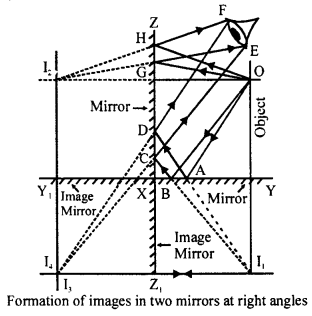Taking normal incidence, I1 and I2 are the images formed in the plane mirror XY and XZ respectively as far behind the mirrors, as point ‘O’ is in front of them.
However, image I1 acts as a virtual object for image mirror XZ1 and forms an image I3. Similarly, image I2 acts as a virtual object for the image mirror XY1 and forms the image I4. The images I3 and I4 overlap to form a very bright image. Thus, on the whole three images are seen. In order to draw two-ray diagrams, from the position FE of the eye, draw two rays meeting at I3,I4 such that these ray intersect the mirror XZ at D and C.
Now draw two rays from point I1 to join C and D intersecting mirror XY at A and B. Join O with A and B.
Similarly, in order to show image I2, draw two rays from I2 to the position of eye FE, such that they intersect at H and G Join H and G to ‘O’ so as to form incident beam.
(b) When two mirrors are parallel to each other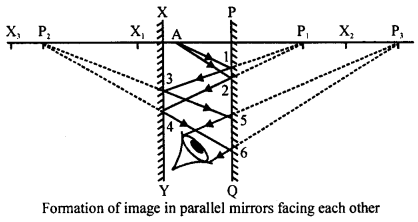Consider two plane mirrors XY and PQ facing each other and ‘A’ as an object situated anywhere between them (See figure).
It is clear that mirror XY forms its image in mirror PQ and vice versa. These images by themsleves will act as image mirror or virtual mirrors.
Let us consider the normal incidence towards the mirror XY for the object A. First of all an image X1 is formed as far behind, as the object is in front of it. This image X1 will fall in front of mirror PQ, and hence, forms an image X2. The image X2 falls in front of mirror XY and hence, forms an image X3. Thus, it continues and infinite images can be formed.
Similarly, taking normal incidence for mirror PQ image P1,P2,P3 etc. are formed.
In order to draw ray diagram, from point A, draw a divergent beam, meeting mirror PQ at points 1 and 2. With P1 as reference point, draw rays 1, 3 and 2, 4 meeting mirror XY. With P3 as reference point draw rays, such that they enter the eye.

Question 10.
Why are infinite images not seen when two plane mirrors are facing each other?
Infinite images are not seen when two plane mirrors are facing each other because :

1. After every successive reflection, some amount of light energy is absorbed. Thus luminosity of image goes on decreasing, till they are no longer visible.
2. As the distance of images from the eye goes on increasing, it is unable to resolve far off images.

Question 11.

(a) State four characteristics of the image formed in a plane mirror.
(b) State three ways in which the image formed in a plane mirror differs from the image formed in a pin hole camera.

(a) Characteristics of Image formed by a Plane Mirror.

1. Image is of the same size as that of object.
2. Image is laterally inverted.
3. It is upright.
4. It is virtual.
5. Image formed is as far behind the mirror as the object infront of the mirror.

(b) Image formed by plane mirror :

1. Image formed by plane mirror is virtual.
2. It can not be obtained on the screen.
3. Size of image is same as that of the object.
4. Image appears to be inverted only on the vertical axis.

Image formed by Pinhole camera :

1. Image formed by pinhole camera is real.
2. It can be obtained on the screen.
3. Size of the image is smaller than the object.
4. Image appears to be inverted on both the vertical axis and horizontal axis.

Question 12.
(a) What should be the minimum size of a plane mirror, so that a person 182 cm high can see himself completely?
(b) A boy stands 4 m away from plane mirror. If the boy moves 1/2 m towards mirror, what is now the distance between the boy and his image? Give a reason for your answer.
(a) Size (height) of a person = 182 cm
We know to see the full length image of a person, we need a plane mirror of size as half of the size of the person.
So, size of plane mirror required = Height of person/2 = 182/2 = 91 cm
(b) Distance of boy from the plane mirror = u = 4 m When boy moves 1/2 m towards the mirror.
Then, distance between boy and mirror = 4 – 1/2 = 7/2 m
We know, in a plane mirror, the image formed is as behind the mirror as the object is in front of it.
So, distance between the mirror and image of boy = 7/2 m
Distance between the boy and his image
= Distance of boy from mirror + Distance of image of boy from mirror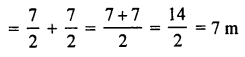Question 13.
State four uses of a plane mirror.
Four uses of plane mirror are :

1. Plane mirrors are used in construction of reflecting periscope.
2.  They are used as looking glass.
3. They are used in solar cookers for reflecting the rays of the sun into the cooker.
4. They are used for signalling purpose.

Question 14.

(a) Draw a neat diagram of reflecting periscope.

(a)(b) Advantages of Reflecting Periscope :

1. It is used to see above the head of crowds.
2. It is used by soldiers in trench warfare.

1. The final images is not brightly illuminated as light energy is absorbed due to two successive reflections.
2. Any deposition of moisture of dust on the mirror reduces the reflection almost to nil, and hence, the periscope cannot be used in places where there is a lot of dust or moisture.

Question 15.
What must be the minimum length of a plane mirror in which a person can see himself full length? Draw a diagram to justify your answer. Does the distance of person from the mirror affect the above answer?
Minimum Height Of Plane Mirror Required For A Person To See Full Length Consider a person AB, such that A represents the highest point on his head, and B the lowest point on the foot such that E is the fixed eye level (see figure). The person will be able to see every part of his body if the can see points A and B. Let MN be the minimum length of mirror fixed on the wall, such that the rays AM and BN, after reflection, reach the eye of person, thereby forming an image A1B1 when produced backward.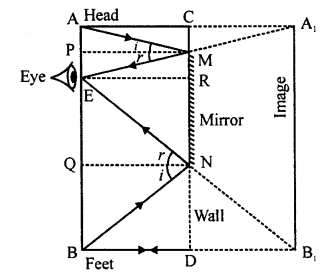In ∆AEA1, CM is parallel to AE and C is the mid-point of AA1. M is the mid-point of A1E.
Similarly, in ∆BEB1, ND is parallel to BE and D is the mid-point of BB1
∴ N in the mid-point of B1E.
Now in ∆A1B1E, M is mid-point of A1E and N is mid-point of B1E.
∴ MN is paralle and half of A1B1
But, A1B1 = AB
So, MN = 1/2 AB.
Thus, in order to see full length, a person requires a plane mirror which is half his own height. This relation is true for any distance of object from plane mirror.

Question 16.
An insect is sitting in front of a plane mirror at a distance of one metre from it.

1. Where is the image of insect formed?
2. What is the distance between insect and its image?
3. State any two characteristics of image formed in a plane mirror.

(i) Distance of insect from plane mirror = u = 1 m
We know in case of plane mirror, distance of object from plane mirror is equal to distance of image of the object from the plane mirror.
∴Distance of the image of insect from plane mirror = v = u – 1 m
(ii) Distance between insect and its image = u + v = 1 + 1 = 2 m
(iii) Characteristics of the image formed by a plane mirror :

(a) Image formed is virtual.
(b) Image formed is erect.
(c) Image formed is of same size as that of the object.

Question 17.
(i) Draw a diagram to show reflection of a ray of light using plane mirror. In the diagram label the incident ray, the reflected ray, the normal, the angle of incidence and angle of reflection. (ii) State the laws of reflection.

1. See Q. No. (2) of Exercise (1).
2. See Q. No. (3) of Exercise (1).

Question 18.
(i) Parallel rays are incident :

1. on regular surface and
2. on irregular surface. In what respect do reflected rays in (1) differ from those of (2)?

(ii) Write down four characteristics of image formed in a plane mirror.
(i) Parallel rays of light after reflection from a regular surface . goes in a particular direction while after reflection from irregular surface go in different directions.
(ii) Characteristics of Image formed by a Plane Mirror.

(a) Image is of the same size as that of object.
(b) Image is laterally inverted.
(c) It is upright.
(d) It is virtual.
(e) Image formed is as far behind the mirror as the object in front of the mirror.

Question 19.
How many images will be formed when an object is placed between two parallel plane mirrors with their reflecting surfaces facing each other? Why do more distant images appear fainter?
Angle between two mirrors facing each other = θ = 0°So, infinite number of images are formed when an object is placed between two parallel plane mirrors with their reflecting surfaces facing each other.
More distant images appear fainter when two plane mirror with their reflecting surfaces facing each other because,
After every successive reflection, some amount of light energy is absorbed. Thus luminosity of images goes on decreasing and hence they appear fainter.

Question 20.

(a) Write down the letters of the word ‘POLEX’ as seen in a plane mirror, held parallel to the plane of this paper.
(b) Distinguish between real and virtual image.

(a)(b)
Real image :

1. Can be taken on the screen.
2. It is inverted.
3. It is formed when light rays after reflection (or refraction) actually meet.

Virtual image :

1. Cannot be taken on screen.
2. It is erect.
3. It is formed when rays of light after reflection (or refraction) appear to meet.

Question 21.

(a) Describe the principle of simple periscope through an outline ray diagram. Give one of its uses.
(b) Draw diagrams to show difference between regular and irregular reflection.

(a) Simple periscope is based upon the principle of reflection. It consists of a cardboard or wooden tube, bent twice at right angles and is provided with two openings as shown in figure. Two plane mirrors are fixed at the bends of the tube at an angle 45° to the framework, such that the mirrors face each other. The tube is completely blackened from inside to avoid any reflection from its sides.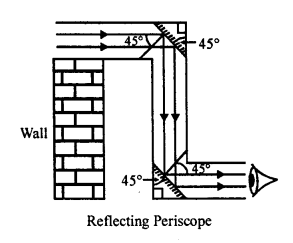The parallel rays coming from an object at a higher plane, strike the plane mirror at an angle of 45° and hence are reflected through an angle of 45°.
These reflected rays strike the second mirror at an angle of 45° and hence are further reflected through an angle of 45°. These reflected rays on reaching the eye form the image on retina.
Use of periscope : It is used by soldiers in trench warfare.
(b)Question 22.
An object is placed 2 cm from a plane mirror. If the object is moved by 1 cm towards the mirror, what will be the distance between the object and its new image?
Distance of the object from plane mirror = 2 cm
If object is moved by 1 cm towards the mirror, then distance between the object and plane mirror = u = 1 cm
We know in case of plane mirror,
Distance of object from plane mirror = Distance of the image of the object from plane mirror
⇒ v = u = 1 cm
∴ Distance between its object and new image = u + v = 1 + 1 = 2 cm

Unit II

Practice Problem 1

Question 1.
An object 3 cm high produces a real image 4.5 cm high, when placed at a distance of 20 cm from a concave mirror. Calculate :

1. the position of image
2. focal length of the concave mirror.

Size of object = ho = 3 cm
Size of image = h1 = -4.5 cm [Image formed is real]
Distance of the object from the concave mirror = u = – 20 cm
Distance of the image from the concave mirror = v = ?
Focal length of the concave mirror = f = ?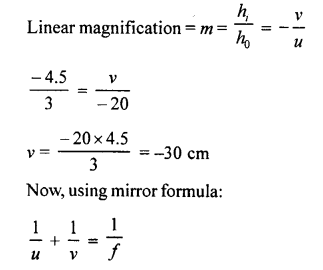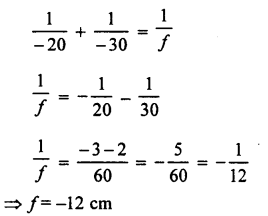Practice Problem 2

Question 1.
An object 1.5 cm high when placed in front of a concave . mirror, produces a virtual image 3 cm high. If the object is placed at a distance of 6 cm from the pole of the mirror, calculate :

1. the position of the image
2. the focal length of the mirror.Question 2.
A converging mirror, forms a three times magnified virtual image when an object is placed at a distance of 8 cm from it. Calculate :

1. the position of the image
2. the focal length of the mirror.

Let size of the object = ho = x
Size of image = h1= +3 x (Virtual image)
Distance of the object from the mirror = u = -8 cm
Distance of the image from the mirror = v = ?
Focal length of the mirror = f = ?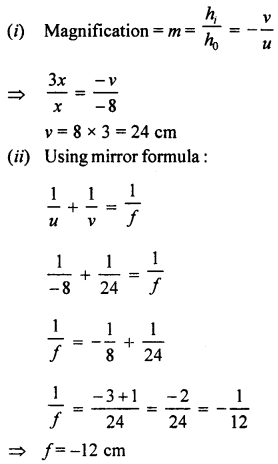Practice Problems 3

Question 1.
An object 5 cm high forms a virtual image of 1.25 cm high, when placed in front of a convex mirror at a distance of 24 cm. Calculate :

1. the position of the image
2. the focal length of the convex mirror.

Size of the object = h0 = 5 cm
Size of the image = h1 = +1.25 cm
Distance of the object from mirror = u = – 24 cm
Distance of the image from the mirror = v = ?
Focal length of the concave mirror = f = ?Question 2.
An object forms a virtual image which is 1/8th of the size of the object. If the object is placed at a distance of 40 cm from the convex mirror, calculate :

1. the position of the image
2. the focal length of the convex mirror.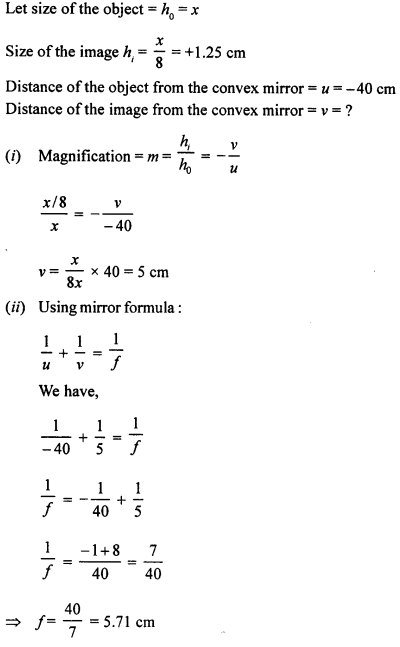Exercise 2

(A) Objective Questions

Multiple Choice Questions.
Select the correct option:

1. A concave mirror is made by cutting a portion of a hollow glass sphere of radius 30 cm. The focal length of the concave mirror is :
(a) 24 cm
(b) 12 cm
(c) 15 cm
(d) 60 cm
Ans. (c) 15 cm
Explanation :
Radius of curvature = R = 30 cm2. A mirror forms a virtual image (diminished) of an object, whatever be the positiion of object :
(a) it must be a concave mirror
(b) it must be a convex mirror
(c) it must be a plane mirror
(d) it may be (b) or (c) or both
Ans. (b) it must be a convex mirror

3. A ray of light is incident on a concave mirror. If it is parallel to principal axis, the reflected ray will :
(a) pass through its principal focus
(b) pass through its centre of curvature
(c) pass through its pole
(d) retraces its path
Ans. (a) pass through its principal focus

4. If an incident ray passes through the centre of curvature of a spherical mirror, the reflected ray will :
(a) pass through its pole
(b) retraces its path
(c) pass through its focus
(d) be parallel to principal axis
Ans. (b) retraces its path

5. In case of concave mirror, the minimum distance between an object and its real image is :
(a) f
(b) 2f
(c) 4f
(d) zero
Ans. (d) zero

6. Looking into a mirror one finds her image diminished, the mirror is :
(a) concave
(b) convex
(c) cylindrical
(d) parabolic
Ans. (b) convex

7. Which mirror is used in periscope?
(a) Convex mirror
(b) Concave mirror
(c) Plane mirror
(d) Parabolic mirror
Ans. (c) Plane mirror

(B) Subjective Questions

Question 1.
Define the following terms :

1. spherical mirror
2. convex mirror
3. concave mirror

1. Spherical mirror : “A mirror which is made from a part of a hollow sphere is called Spherical Mirror.
2. Convex mirror : “A mirror made by silvering the inner surface such that reflection takes place from the bulging surface” is called Convex Mirror.
Centre of curvature is towards the silvered surface.
3. Concave mirror : “A mirror made by silvering the outer or the bulging surface such that the reflection takes place from the concave surface.” Centre of curvature is towards the reflecting surface.

Question 2.
Define the following terms in relation to concave mirror.

1. Pole
2. Centre of curvature
3. Principal axis
4. Principal focus
5. Focal length
7. Aperture

1. Pole : Pole “is the mid-point of the mirror”.
2. Centre of curvature : The centre of hollow sphere of which the mirror forms a part, is called centre of curvature.
3. Principal axis : An imaginary line passing through the pole and the centre of curvature of a spherical mirror is called principal axis
4. Principal focus : It is a point on the principal axis, where a beam of light, parallel to principal axis, after reflection actually meet.
5. Focal length : The linear distance between the pole and the principal focus is called focal length.
6. Radius of curvature : The linear distance between the pole and the centre of curvature is called radius of curvature.
7. Aperture : The diameter of a spherical mirror is called its aperture.

Question 3.

(a) Define the term principal focus in case of convex mirror. Draw a convex mirror and show its principal focus and focal length clearly.
(b) What is the relation between focal length and radius of curvature of a concave mirror?

(a) Focal length is the distance between pole (P) and focus (F)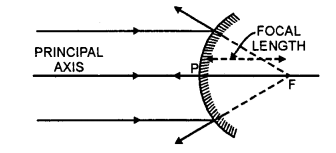(F) Focus is a point on principal axis where rays of light appear to meet.
(b) Focal length of a spherical mirror is equal to half of the radius of curvature of spherical mirror.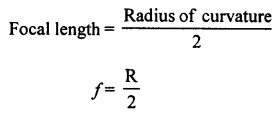Question 4.

(a) What do you understand by the term real image?
(b) What type of mirror is used to obtain a real image?
(c) Does the mirror named by your form real image for all locations? Give reason for your answer.
(d) Is real image always inverted?

(a) Real image : When rays of light after reflection or refraction actually meet at some other point” the image is real.
(b) Concave mirror.
(c) No, this mirror does not give real image of the object that lies between principal focus and pole.
(d) Yes. Real image is always ihverted.

Question 5.
Copy the figure. By taking two rays from point A, show the formation of image. State four characteristics of the image.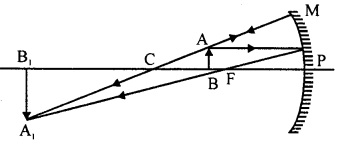Characteristics of the image :

1. Image formed is real.
2. Image formed is inverted.
3. Image formed is enlarged.
4. Image is formed beyond centre of curvature in front of concave mirror.

Question 6.
Draw a neat two ray diagram to illustrate how a concave mirror is used as a shaving mirror.
We know that when an object is placed between P and F of a concave mirror, it forms a virtual and enlarged image. Thus, by using concave mirror we can have a proper shave, as the tiny hairs are clearly visible.Question 7.
Copy the figure. By taking two rays from point A, show the formation of image. State four characteristics of the image.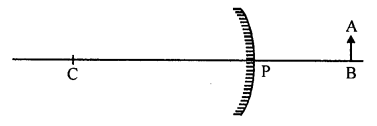Characteristics of image :

1. Image formed is virutal.
2. Image formed is erect.
3. Image formed is diminished.
4. Image is always formed between pole and principal focus, behind the convex mirror.

Question 8.
Why do automobile drivers prefer convex mirror as a rear vew mirror? Illustrate your answer.
A convex mirror always forms a small and upright image between pole and focus. That means in small area of mirror driver can see all the traffic coming from behind.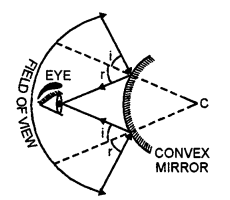Question 9.
Give two uses of

1. convex mirror
2. concave mirror.

(i) Two uses of convex mirror are :

(a) It is used as a rear view mirror in automobile to see the traffic behind.
(b) It is used as reflector for street light bulbs.

(ii) Two uses of concave mirror are :

(a) Concave mirror is used as A Reflector in head lights of cars and in search light. The source of light (bulb) is placed at the principal focus and the reflector forms parallel beam of light.
(b) For doctors to examine throat, ear, nose and eyes, light is focused with the help of concave mirror.

Question 10.
You are provided a convex mirror, a concave mirror and a plane mirror. How will you distinguish between them, without touching or using any other apparatus?
We can identify convex mirror, concave mirror and a plane mirror by looking at them one by one.

1. If the size of the image of an object is of the same size as that of the object, then that mirror is a plane mirror.
2. If the size of image of an object increases as the object is brought closer to the mirror and size of the image of the object decreases when the object is taken away from the mirror, then that mirror is a concave mirror.
3. If the size of the iamge of an object remains diminished, either the object is moved away from the mirror or moved towards the mirror, then that mirror is a convex mirror.

Question 11.
Compare the characteristics of an image formed by a convex mirror and a concave mirror, when object is beyond centre of curvature, but not at infinity.
Characteristics of the image formed by concave mirror when object is beyond centre of curvature, but not at infinity are :

1. Image formed is real.
2. Image formed is inverted.
3. Image formed is diminished.
4. Image is formed between centre of curvature (C) and principal focus (F), in front of the concave mirror.

Characteristics of the image formed by convex mirror when object is beyond centre of curvature, but not at infinity are :

1. Image formed is virutal.
2. Image formed is erect.
3. Image formed is diminished.
4. Image is always formed between pole and principal focus and behind the convex mirror.

Question 12.

1. Why does a driver use a convex mirror as a rear view mirror?

See Q. No. 8 of Exercise (2).

Question 13.

1. What is a real image?
2. What type of mirror is used to obtain a real image-of an object?
3. Does the mirror named by you above give real images for all locations of object?

1. Real image : When the rays of light diverging from a point, after reflection of refraction, actually converge at some point, then that point is the real image of the object.
2. Concave mirror is used to obtain the real image of an object.
3. Concave mirror can not give real image for all the locations of object.

Question 14.
In the figure is shown a concave mirror. A is a point on the principal axis. If an object O is kept at A, image is formed on A itself. Copy the diagram. Draw the image in the diagram. Is the image real or virtual?Measure the distance PA and write it in the diagram. What is the distance PA called?
Mark a point B on the principal axis, at which, if a point source of light is kept, the rays travel parallel to principal axis after reflection from M. What is point B called?
As the object is placed at A and its image is also formed at A, so object must be at centre of curvature (C).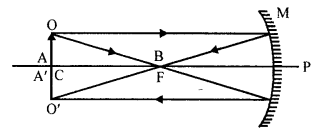PA is called radius of curvature and on measuring PA = 4.8 cm. Point B marked on principal axis is called principal focus.

Question 15.
An object OA is placed on the principal axis of a concave mirror as shown in the figure. Copy and complete the diagram to show the formation of image.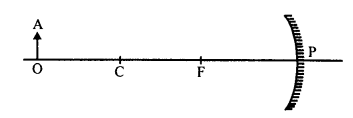Question 16.
Copy the figure and complete it, by drawing two rays to show the formation of the image of the object AB. State the size, position and nature of image formed.